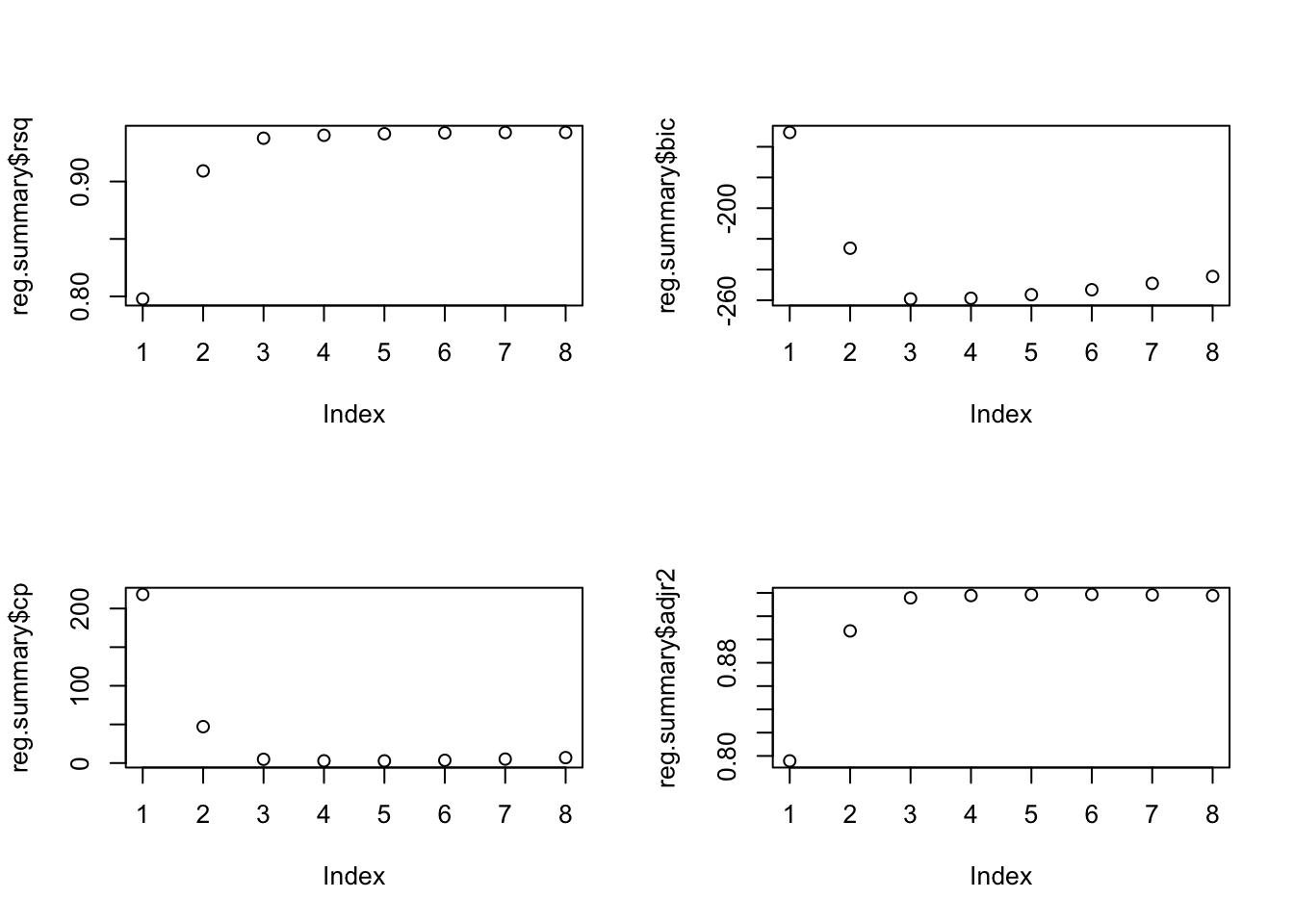ISLR Home

# Question

p262

In this exercise, we will generate simulated data, and will then use this data to perform best subset selection.

1. Use the rnorm() function to generate a predictor X of length n = 100, as well as a noise vector ε of length n = 100.

2. Generate a response vector Y of length n = 100 according to the model Y = β0 +β1X +β2X2 +β3X3 +ε, where β0, β1, β2, and β3 are constants of your choice.

3. Use the regsubsets() function to perform best subset selection in order to choose the best model containing the predictors X, X2, . . . , X10. What is the best model obtained according to Cp, BIC, and adjusted R2? Show some plots to provide evidence for your answer, and report the coefficients of the best model ob- tained. Note you will need to use the data.frame() function to create a single data set containing both X and Y . 2b setting.

4. Repeat (c), using forward stepwise selection and also using back- wards stepwise selection. How does your answer compare to the results in (c)?

5. Now fit a lasso model to the simulated data, again using X,X2, . . . , X 10 as predictors. Use cross-validation to select the optimal value of λ. Create plots of the cross-validation error as a function of λ. Report the resulting coefficient estimates, and discuss the results obtained.

6. Now generate a response vector Y according to the model Y = β0 + β7X7 + ε, and perform best subset selection and the lasso. Discuss the results obtained

``library(ISLR)``

# 8a Random x vector

``````set.seed(112)
x <- rnorm(100)
epsilon <- rnorm(100)``````

# 8b betas

``````beta0 = 1
beta1 = 1
beta2 = 1
beta3 = 1

beta0 = 3
beta1 = 2
beta2 = -3
beta3 = 0.3

Y <- beta0 + beta1 * x + beta2 * x^2 + beta3 * x^3 + epsilon``````

# 8c

``````library(leaps)
regfit.full=regsubsets(Y~., poly(x, 10))
reg.summary=summary(regfit.full)

names(reg.summary)``````
``##  "which"  "rsq"    "rss"    "adjr2"  "cp"     "bic"    "outmat" "obj"``
``reg.summary\$rsq``
``````##  0.7978113 0.9092099 0.9376857 0.9402457 0.9415817 0.9423889 0.9426592
##  0.9427198``````
``reg.summary\$bic``
``````##  -150.6451 -226.1050 -259.1357 -258.7256 -256.3816 -253.1678 -249.0329
##  -244.5335``````
``reg.summary\$cp``
``````##  218.238194  47.104395   4.847869   2.869052   2.792670   3.538211   5.118088
##    7.023931``````
``reg.summary\$adjr2``
``````##  0.7957482 0.9073380 0.9357383 0.9377298 0.9384744 0.9386720 0.9382963
##  0.9376842``````
``````par(mfrow=c(2,2))
plot(reg.summary\$rsq)
plot(reg.summary\$bic)
plot(reg.summary\$cp)# 8d

``````regfit.full=regsubsets(Y~., poly(x, 10), method = "forward")
reg.summary=summary(regfit.full)

names(reg.summary)``````
``##  "which"  "rsq"    "rss"    "adjr2"  "cp"     "bic"    "outmat" "obj"``
``reg.summary\$rsq``
``````##  0.7978113 0.9092099 0.9376857 0.9402457 0.9415817 0.9423889 0.9426592
##  0.9427198``````
``reg.summary\$bic``
``````##  -150.6451 -226.1050 -259.1357 -258.7256 -256.3816 -253.1678 -249.0329
##  -244.5335``````
``reg.summary\$cp``
``````##  218.238194  47.104395   4.847869   2.869052   2.792670   3.538211   5.118088
##    7.023931``````
``reg.summary\$adjr2``
``````##  0.7957482 0.9073380 0.9357383 0.9377298 0.9384744 0.9386720 0.9382963
##  0.9376842``````
``````par(mfrow=c(2,2))
plot(reg.summary\$rsq)
plot(reg.summary\$bic)
plot(reg.summary\$cp)

regfit.full=regsubsets(Y~., poly(x, 10), method = "backward")
reg.summary=summary(regfit.full)

names(reg.summary)``````
``##  "which"  "rsq"    "rss"    "adjr2"  "cp"     "bic"    "outmat" "obj"``
``reg.summary\$rsq``
``````##  0.7978113 0.9092099 0.9376857 0.9402457 0.9415817 0.9423889 0.9426592
##  0.9427198``````
``reg.summary\$bic``
``````##  -150.6451 -226.1050 -259.1357 -258.7256 -256.3816 -253.1678 -249.0329
##  -244.5335``````
``reg.summary\$cp``
``````##  218.238194  47.104395   4.847869   2.869052   2.792670   3.538211   5.118088
##    7.023931``````
``reg.summary\$adjr2``
``````##  0.7957482 0.9073380 0.9357383 0.9377298 0.9384744 0.9386720 0.9382963
##  0.9376842``````
``````par(mfrow=c(2,2))
plot(reg.summary\$rsq)
plot(reg.summary\$bic)
plot(reg.summary\$cp)# 8e

## STUDY!

``library(glmnet)``
``## Loading required package: Matrix``
``## Loaded glmnet 4.0-2``
``````set.seed(1)

df = data.frame(x = x,
y = Y)
# train = sample(1:nrow(df), nrow(df)*.7)

x = model.matrix(y ~ poly(x, 10, raw = T), data = df)[, -1]
mod.lasso = cv.glmnet(x, Y, alpha = 1)

best.lambda = mod.lasso\$lambda.min
best.lambda``````
``##  0.01324949``
``plot(mod.lasso)``